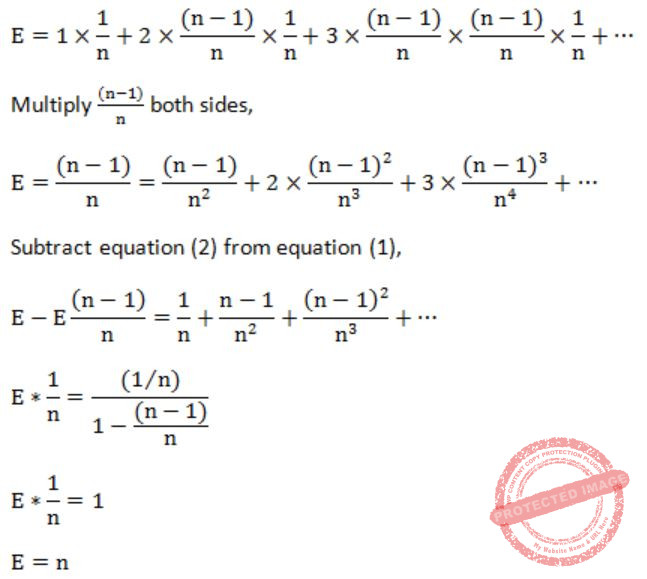## Searching

 Question 1

Let A be an array of 31 numbers consisting of a sequence of 0’s followed by a sequence of 1’s. The problem is to find the smallest index i such that A[i] is 1 by probing the minimum number of locations in A. The worst case number of probes performed by an optimal algorithm is _________.

 A 5 B 6 C 7 D 8
Algorithms       Searching       GATE 2017 [Set-1]       Video-Explanation
Question 1 Explanation:
→ If we apply binary search to find the first occurrence of 1 in the list, it will give us the smallest index i where 1 is stored.
→ As in this array sequence of 0’s is followed by sequence of 1’s, the array is sorted. We can apply binary search directly without sorting it.
So number of probes = ceil(log2 31) = 4.954196310386876
⇒ here we are using ceiling so it becomes 5
 Question 2

Consider the C function given below. Assume that the array listA contains n (> 0) elements, sorted in ascending order.

```int ProcessArray(int *listA, int x, int n)
{
int i, j, k;
i = 0;
j = n-1;
do
{
k = (i+j)/2;
if (x <= listA[k])
j = k-1;
if (listA[k] <= x)
i = k+1;
}
while (i <= j);
if (listA[k] == x)
return(k);
else
return -1;
}```

Which one of the following statements about the function ProcessArray is CORRECT?

 A It will run into an infinite loop when x is not in listA. B It is an implementation of binary search. C It will always find the maximum element in listA. D It will return −1 even when x is present in listA.
Algorithms       Searching       GATE 2014 [Set-3]
Question 2 Explanation:
From the above code, we can identify
k = (i+j)/2;
where k keeps track of current middle element & i, j keeps track of left & right children of current subarray.
So it is an implementation of Binary search.
 Question 3

Consider the following algorithm for searching for a given number x in an unsorted array A[1...n] having n distinct values:

```        1. Choose an i uniformly at random from 1...n;
2. If A[i]=x then Stop else Goto 1; ```

Assuming that x is present in A, what is the expected number of comparisons made by the algorithm before it terminates?

 A n B n-1 C 2n D n/2
Algorithms        Searching       GATE 2002
Question 3 Explanation:Question 4

Which one of the following statements is false?

 A Optimal binary search tree construction can be performed efficiently using dynamic programming. B Breadth-first search cannot be used to find connected components of a graph. C Given the prefix and postfix walks over a binary tree, the binary tree cannot be uniquely constructed. D Depth-first search can be used to find connected components of a graph.
Algorithms       Searching       GATE 1994
Question 4 Explanation:
In BFS algorithm, we can randomly select a source vertex and then run, after that whether we need to check distance to each and every vertex from source is still infinite (or) not. If we find any vertex having infinite distance then the graph is not connected.
 Question 5
The time taken by binary search algorithm to search a key in a sorted array of n elements is
 A O ( log2n ) B O ( n ) C O ( n log2n ) D O ( n2 )
Algorithms       Searching       ISRO-2007
Question 5 Explanation:
Search a sorted array by repeatedly dividing the search interval in half. Begin with an interval covering the whole array. If the value of the search key is less than the item in the middle of the interval, narrow the interval to the lower half. Otherwise narrow it to the upper half. Repeatedly check until the value is found or the interval is empty. It takes a maximum of log(n) searches to search an element from the sorted array.
 Question 6
The recurrence relation that arises in relation with the complexity of binary search is:
 A T(n)=2T(n/2)+k , where k is constant B T(n)=T(n/2) +k, where k is constant C T(n)=T(n/2)+logn D T(n)=T(n/2)+n
Algorithms       Searching       ISRO-2017 May       Video-Explanation
Question 6 Explanation:
Binary search in a sorted array
The time to search in an array of ‘n’ elements is equal to the time to search in an array of n/2 elements plus k comparison.
T(n)=T(n/2)+k // k is constant
 Question 7
The time required to search an element in a linked list of length n is
 A O(log n) B O(n) C O(1) D (n2)
Data-Structures       Searching       ISRO CS 2008
Question 7 Explanation:
In the worst case, the element to be searched has to be compared with all elements of linked list. It will take O(n) time to search the element.
There are 7 questions to complete.

Register Now